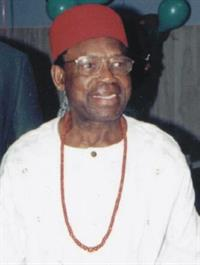Chiki Obi

Personal

 First Name: Chiki
 Last Name: Obi

Biography

 Chike Obi, with James Ezeilo and Adegoke Olubummo, was one of a trio of black mathematicians who pioneered modern mathematics research in Nigeria. Dr. Obi's early research dealt mainly with the question of the existence of periodic solutions of non-linear ordinary differential equations. He successfully used the perturbation technique and several of his publications greatly helped to stimulate research interest in this subject throughout the world and have. in fact, become classics in the literature. Recently Dr. Obi has claimed a short (10 pages) proof of Fermat's Last Theorem (see news articles), the result whose 1996 solution by Andrew Wiles set the mathematics world on fire. For a price, you can order Obi's article at the web page Algebras Groups and Geometry or from HADRONIC PRESS 35246 US 19 North, # 115 Palm Harbor, FL 34684, USA Fax +1-813-934 9275 However, a Mathematical Reviews' article essentially claims Obi's proof is a known false proof and hence no solution. Articles about Professor Chike: J. Nigerian Mathematical Society, University of Ibadan, Department of Mathematics, Ibadan, 1992. pp. i--iv and 1--114. Animalu, A. O. E., Professor Chike Obi [b. 1921]. Special issue in honour of Professor Chike Obi. J. Nigerian Math. Soc. 11(1992), no. 1, i--iv. Research Papers Obi, C. Fermat's last theorem. Algebras Groups Geom. 15 (1998), no. 3, 289--298. Obi, Chike A study of the boundedness of the solutions of non-linear differential equations of order $2$. Special issue in honour of Professor James O. C. Ezeilo. J. Nigerian Math. Soc. 11 (1992), no. 3, 29--43. Obi, Chike Erratum: The boundedness of solutions of nonlineardifferential equations of even order $2s$ [J. Differential Equations {\bf 58} (1985), no. 3, 428--446]. J. Differential Equations 75 (1988), no. 1, 186. 34C11 Obi, Chike A system of order $2$ in which small subharmonics and large subharmonics coexist. Proc. Roy. Soc. Edinburgh Sect. A 108 (1988), no. 1-2, 35--44. Obi, Chike The boundedness of solutions of nonlinear differential equations of even order $2s$. J. Differential Equations 58(1985), no. 3, 428--446. Obi, Chike Analytical theory of nonlinear oscillations. X . Some classes of equations $\ddot x+g(x)=0$ with no finite Fourier series solution. Atti Accad. Naz. Lincei Rend. Cl. Sci. Fis. Mat. Natur. (8) 67(1979), no. 1-2, 53--56 (1980). Obi, Chike Analytical theory of nonlinear oscillations. IX . Limit oscillations and unbounded solutions of equations of the second order. Ann. Mat. Pura Appl. (4) 120(1979), 139--157. Obi, Chike Analytical theory of non-linear oscillations. VIII . Second-order conservative systems whose solutions are all oscillating with the least period $2\pi$. Ann. Mat. Pura Appl. (4) 117 (1978), 339--347. Obi, Chike Analytical theory of nonlinear oscillations. IV . The periodic oscillations of the equation $x-\epsilon (1-x\sp{2n+2})x+x\sp{2n+1}=\epsilon a {\rm cos} \omega t, a>0, \omega >0$ independent of $\epsilon$. SIAM J. Appl. Math. 31 (1976), no. 2, 345--357. Obi, Chike Analytical theory of nonlinear oscillations. II. Small periodic oscillations of equations of the second order. SIAM J. Appl. Math. 31 (1976), no. 2, 334--344. Obi, Chike Analytical theory of nonlinear oscillations. VII. The periods of the periodic solutions of the equation $x+g(x)=0$. J. Math. Anal. Appl. 55(1976), no. 2, 295--301. Obi, Chike Analytical theory of non-linear oscillations. I. An existence theorem for periodic oscillations of equations of the second order. Proc. Cambridge Philos. Soc. 76 (1974), 285--296. Obi, Chike Uniformly almost periodic solutions of nonlinear differential equations of the second order. I. General exposition. Proc. Cambridge Philos. Soc. 51 (1955), 604--613. Obi, Chike Researches on the equation (E) $\ddot x+(\epsilon\sb 1+\epsilon\sb 2x)\dot x+x+\epsilon\sb 3x\sp 2=0$. Proc. Cambridge Philos. Soc. 50, (1954). 26--32. Obi, Chike Periodic solutions of non-linear differential equations of order $2n$. J. London Math. Soc. 28, (1953). 163--171. Obi, Chike A non-linear differential equation of the second order with periodic solutions whose associated limit cycles are algebraic curves. J. London Math. Soc. 28, (1953). 356--360. Obi, Chike Periodic solutions of non-linear differential equations of the second order. V. Proc. Cambridge Philos. Soc. 47, (1951). 752--755. Obi, Chike Periodic solutions of non-linear differential equations of the second order. IV. Proc. Cambridge Philos. Soc. 47, (1951). 741--751. Obi, Chike Subharmonic solutions of non-linear differential equations of the second order. J. London Math. Soc. 25, (1950). 217--226.   We had help with this web page from Alex Animalu. References: Maematical Reviews